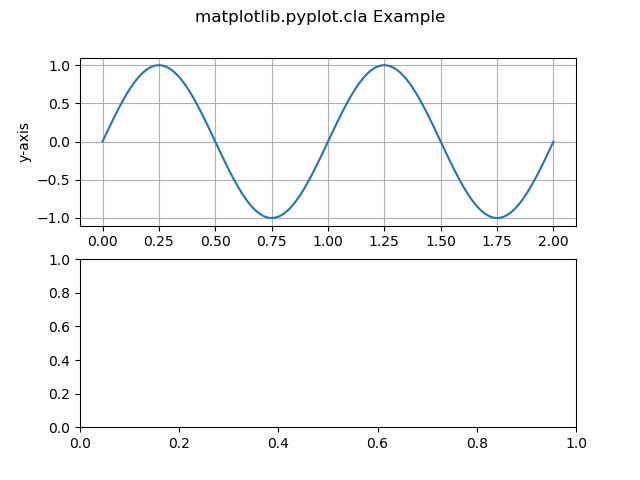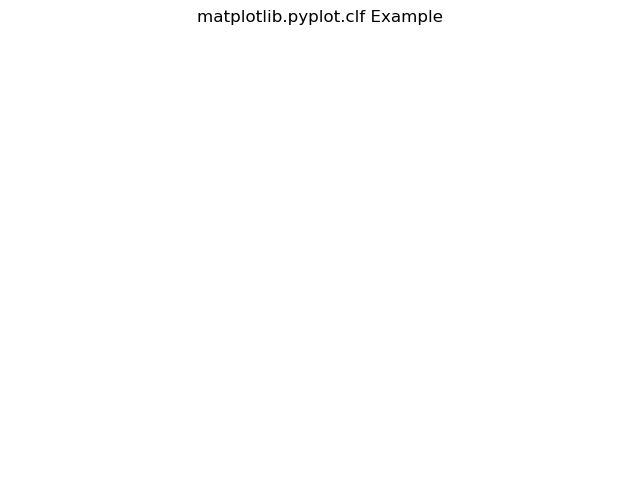# Difference Between cla(), clf() and close() Methods in Matplotlib

• Last Updated : 12 Sep, 2022

Matplotlib is a library in Python. It is a mathematical extension of the Numpy Library. It is a comprehensive library for creating static, animated, and interactive visualizations in Python. Pyplot is a state-based interface to a Matplotlib module. The Pyplot can create many types of plots such as line graphs, bar graphs, histograms, etc. The cla(), clf() and close() are different methods and functions of Matplotlib.

## What is cla() in Matplotlib

This cla()method in the Pyplot module of the Matplotlib library is used to clear the current axes.

## Python3

 `import` `numpy as np``import` `matplotlib.pyplot as plt` `t ``=` `np.linspace(``0.0``, ``2.0``, ``401``)``s ``=` `np.sin(``2` `*` `np.pi ``*` `t)` `fig, [ax, ax1] ``=` `plt.subplots(``2``, ``1``)` `ax.set_ylabel(``'y-axis'``)``ax.plot(t, s)``ax.grid(``True``)` `ax1.set_ylabel(``'y-axis'``)``ax1.set_xlabel(``'x-axis'``)``ax1.plot(t, s)``ax1.grid(``True``)``# Function call``ax1.cla()` `fig.suptitle(``'matplotlib.pyplot.cla Example'``)``plt.show()`

Output:The subplot ax1 content is cleared.

## What is clf() in Matplotlib

The clf() method in the Pyplot module of the Matplotlib library is used to clear the entire current figure. It even clears the subplot. It leaves the window space open so that it can be reused by other plots.

## Python3

 `import` `numpy as np``import` `matplotlib.pyplot as plt` `t ``=` `np.linspace(``0.0``, ``2.0``, ``201``)``s ``=` `np.sin(``2` `*` `np.pi ``*` `t)` `fig, [ax, ax1] ``=` `plt.subplots(``2``, ``1``)` `ax.set_ylabel(``'y-axis'``)``ax.plot(t, s)``ax.grid(``True``)` `ax1.set_ylabel(``'y-axis'``)``ax1.set_xlabel(``'x-axis'``)``ax1.plot(t, s)``ax1.grid(``True``)` `# Func. call``plt.clf()` `fig.suptitle(``'matplotlib.pyplot.clf Example'``)``plt.show()`

Output:The clf() function cleared the entire figure and only space is left.

## What is close() Matplotlib

The close() method in the Pyplot module of the Matplotlib library is used to close the window of the plot. By default, it closes the current window. Since the window is closed there will no output in this method.

## Python3

 `import` `numpy as np``import` `matplotlib.pyplot as plt` `t ``=` `np.linspace(``0.0``, ``2.0``, ``201``)``s ``=` `np.sin(``2` `*` `np.pi ``*` `t)` `fig, [ax, ax1] ``=` `plt.subplots(``2``, ``1``)` `ax.set_ylabel(``'y-axis'``)``ax.plot(t, s)``ax.grid(``True``)` `ax1.set_ylabel(``'y-axis'``)``ax1.set_xlabel(``'x-axis'``)``ax1.plot(t, s)``ax1.grid(``True``)` `# Function call``plt.close()` `fig.suptitle(``'matplotlib.pyplot.close Example'``)``plt.show()`

## Difference Between cla() vs. clf() vs. close()

My Personal Notes arrow_drop_up